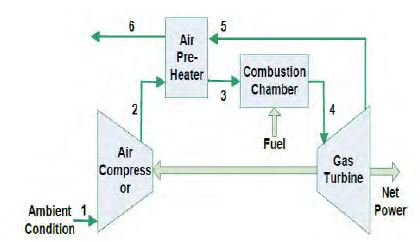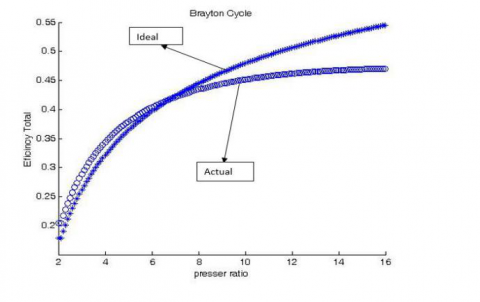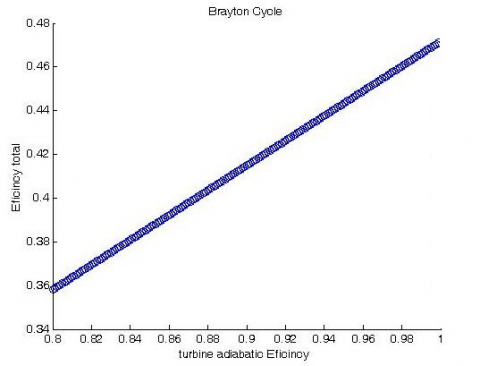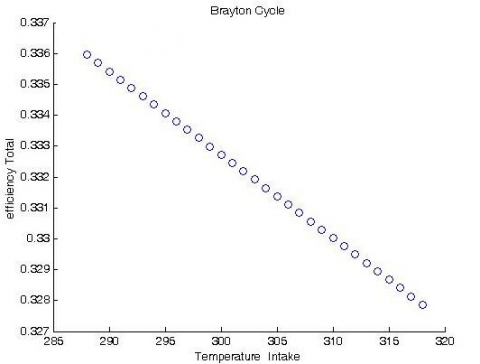# Technical and economical optimization of CHP systems by using gas turbine and energy recovery system

Technical and economical optimization of CHP systems by using gas turbine and energy recovery system

Islamic Azad University Damavand Branch, Damavand, Iran

Department of Chemical Engineering, Payame Noor University (PNU), P.O. Box, 19395-3697 Tehran, Iran

Faculty of Mechanical Engineering, Shahrood University of Technology, Shahrood, Iran

Università degli Studi di Parma, Dipartimento di Ingegneria e Architettura, Parma 43124, Italy

Department of Mechanical Engineering, Lovely Professional University, Phagwara-144411 Punjab, India

Corresponding Author Email:
Page:
286-292
|
DOI:
https://doi.org/10.18280/mmep.050403
23 August 2018
|
Accepted:
1 November 2018
|
Published:
31 December 2018
| Citation

OPEN ACCESS

Abstract:

In this paper, the technical and economic optimization of a CHP system using gas turbine and energy recovery system is performed. The genetic algorithm has been used to optimize the performance of the power plant. Thermodynamic modeling of the CHP was initially performed by applying MATLAB software and the second law efficiency was obtained for the system. The cost of each component was defined as a function. Afterwards, operating and maintenance costs were investigated and the function of overall costs were obtained. Design parameters considered for economic optimization were compressor pressure ratio ( $r_{C}$ ), isentropic compressor efficiency ( $\eta_{\mathrm{c}}$ ), isentropic turbine efficiency (ƞT), combustion inlet temperature (T3) and turbine inlet temperature (T4). The efficiency of the power plant was 36.6% which must be improved by optimization. In order to enhance the efficiency, the flow rate of fuel was optimized which led to the improvement of the efficiency. The optimized efficiency was 48.9%.

Keywords:

technical and economic optimizati, simultaneous production system, gas turbine, energy recycling system

1.Introduction

In recent decades, manufacturers and consumers have planned to reduce their energy-related costs; therefore, CHP systems utilization have been increased. The CHP system, combined heat and power generation, is a synchronous mechanical and thermal energy production using a fuel source in a single system, whose mechanical energy is generally converted into electricity by the generator and the heat dissipation in the sector is mechanically used. This is recovered in order to produce hot water, steam, hot air or for other applications.

The temperature of the exhaust gases from a gas turbine cycle is usually very high (about 500 °C). It is important to use these gases to optimize the gas turbine cycle. In most cases, heat exchangers are used to recover energy from hot- exhaust gases and produce steam.

To exploit these gases in a gas turbine, and basically to optimize any other energy system, the following objectives should be considered as much as possible:

• Highest efficiency of the system

• Lowest total cost

• Lowest energy consumption

• Least harmful environmental and human impacts

• Highest safety

• Highest reliability of continuous and system-free operation.

Several studies have been conducted on the utilization of hot gases exiting turbine in order to achieve higher system efficiency. Sayyaadi and Aminian  conducted a research on the optimization of non-TEMA shell and tube heat exchanger for a pressure booster station by applying genetic algorithm. Sanaye and Hajj-Abdollahi  used multi-objective optimization for a plant fin converter using genetic algorithm. They performed the optimization based on minimization of energy production and capital return and applying FUZZY and LINMAP decision-making tools. In another work, Michal et al.  modeled and developed an approach to optimize the CHP technology operations in a plant in order to maximize profit. Erbay and Goktun  reviewed the design of the recovery turbine with increasing coherent heat, which works based on the Bryton cycle. The aim of this study was achieving high efficiency and small gas turbines. The optimization was done numerically based on obtaining the highest power and the highest power density. Erbay and Goktun concluded that the recovery gas turbine cycle, which increases the heat of the homogeneous system by a secondary combustion chamber, has the highest efficiency among all types of the recovery cycles. In another work done by Chen and Li , power optimization for a renewable cycle of gas turbine was considered. In this study, analytical formulas were obtained to achieve the relationship between output power and total cycle pressure ratio. The purpose of this study was determining the optimal mass flow or optimal pressure drop, which would allow the whole system to operate at the maximum output power. Ponce et al.  focused on single-objective optimization of a shell and tube heat exchanger, by considering geometric characteristics as decision variables, they achieved the goal of reducing the overall annual costs.

Soltani et al.  investigated a gas turbine combined cycle with biomass gasification. The fuels for topping and bottoming cycle were natural gas and various biomass fuels, respectively. The utilized biomass options included wood, paper, paddy husk, and municipal waste . In addition, the systems were examined by entirely using natural gas and coal (in a separate analysis) in order to compare results.  Recently, Freschi et al.  studied tri-generation systems in the food industry and investigated the systems based on environmental and economic aspects. A multi-objective optimization was performed which focused operational costs and greenhouse gases emissions. This enabled the authors to devise various operating strategies for the system. In addition, a sensitivity analysis was also included in this work as the authors recognized the impact the pricing in the economic analysis may have contributed to the final results. Namuli et al.  conducted a study based on net present value (NPV) in order to obtain the optimum herd size which is needed to produce adequate biomass waste to make a CHP system commercially appropriate. The plant was designed with a digester which was utilized convert the manure and food wastes into biogas. The obtained biogas was used in an internal combustion engine to generate electricity and heat. In another study, Wongchanapai et al. , conducted a sensitivity analysis in order to assess the effect of the influential working parameters of a direct-biogas SOFC-micro gas turbine (MGT) hybrid CHP system which has 200 kWe power output capacity.

Assessment of economic feasibility is significantly important in designing CHP systems. Various studies have been conducted in order to economically analyze CHP with different fuels including natural gas, especially working with gas turbines. The performed economic analyses consider both electrical and thermal outputs , system capacity, types of prime mover, and working scenarios , and some of the consider climate zones for investigation . Biogas is one of the renewable energy sources which are used in various industrial activities. Technoeconomic analyses have been performed on CHP systems working with bio-gases as fuel in some studies. For instance, in Ref. 14 various CHP systems are investigated economically ; moreover, some other studies focused on economic and environmental benefits of the CHP systems working with bio-gas . In addition to CHP systems, biogas can be applied in trigeneration systems  which indicates that these fuels are appropriate for several applications.

As mentioned in literature review, there is not any study which has focused on technical and economic optimization of CHP systems working with biogas. In this study, a CHP system working with biogas is investigated and optimized technically and economically. In this system, heat recovery unit is utilized in order to achieve higher efficiency and lower cost. The details of the investigation and the results are represented in following sections.

2.Modeling

2.1 Gas turbine specifications

Most of the gas turbines used in Iran’s power plants are manufactured by Siemens and have the same operating cycle. The turbine tested here has a capacity of 195 mw. The turbine cycle has a reciprocator, the compressed air heated by the exhaust gas from the turbine; afterwards, enters the combustion chamber. Figure 1 shows the general diagram of the cycle and Table 1 shows the assumptions of the problem.

## 1.pngFigure 1. General diagram of gas turbine cycle

Table 1. 195 MW gas turbine specification

 (rp) Compressor pressure ratio 11 (ƞC) Isentropic compressor efficiency 0.85 (ƞT) Isentropic turbine efficiency 0.85 (TC) Entrance temperature to turbine 1640 k (T1) Inlet temperature to compressor 298 k

2.2 Thermodynamic modeling

The overview of the gas turbine cycle with heat exchanger is shown in Fig. 1. The main components of this system are gas turbine (compressors, combustion chambers and turbines) and heat exchangers (recuperators). The recuperator is a cross-flow heat exchanger used to recover heat and optimize energy in industrial units. The recuperator is located at the inlet and outlet sections of the systems in which air acts as a cooling or heating fluid. In these systems, the air recuperator is utilized for heat recovery from the generated waste heat. The compressor and turbine are modeled by considering the adiabatic assumption, taking into account the polytropic efficiency. By calculating the combustion chamber inlet air temperature and the exhaust gases from the combustion chamber, the mass rate of fuel (methane) can be calculated.

2.3 Assumptions

It should be noted that in order to use thermodynamic relations, the following thermodynamic assumptions are considered.

• All the processes in the study are based on steady state model.

• The principles of mixing gas and air are used.

• Fuel utilized in the combustion chamber is methane with LHV = 50000 kj / kg.

• Kinetic potential energy is considered to be insignificant due to its low velocity and height. In addition, the wear and friction are considered to be negligible.

• The waste heat from the combustion chamber is assumed to be 3% of the value of the lower heating value (LHV) of the fuel.

• In the converter, the pressure of the gas side is 3% and 5% on the air side, the pressure drop in the combustion chamber is 5%.

2.4 Equations governing the temperature of the adiabatic flame

The reaction equations for methane are in the form of equation (1)

$\mathrm{CH}+\lambda \times \times 0+\mathrm{N} \rightarrow \lambda \times \mathrm{CO}+\mathrm{HO}+\lambda \times \times \mathrm{N}$     (1)

$x_{1} C H_{4}+x_{2} C_{2} H_{6}+x_{3} C_{3} H_{8}+x_{4} I C_{4} H_{10}+x_{5} n C_{4} H_{10}+x_{6} I C_{5} H_{12}+x_{7} n C_{5} H_{12}$

$+\lambda \times 2 x_{8}\left(O_{2}+3.76 N_{2}\right) \rightarrow x_{9} C O_{2}+x_{10} H_{2} O+\lambda 2 \times 3.76 N_{2}$     (2)

In which is the coefficient of excessive air and . are molecular coefficients of the constituents of natural gas. The governing relations of the adiabatic flame temperature are based on the first law of thermodynamics, which is in the form of equation (3) .

$\sum_{R} n_{R}\left(\overline{h}_{f}^{o}+\Delta \overline{h}\right)_{R} )_{T_{i n}}$=$\sum_{P} n_{P}\left(\overline{h}_{f}+\Delta \overline{h}\right)_{P} )$$T_{\text { flame }} (3) In which is the molar amount of the species participating in the reaction (before combustion) and n_{P} is the molar amount of reactive product species (after combustion). \overline{h}_{f}^{o} is the enthalpy formation at reference temperature and \Delta \overline{\boldsymbol{h}} is the enthalpy difference between temperature T and \boldsymbol{T}_{\boldsymbol{r} e \boldsymbol{f}} reference. The assumptions are as follows:A. Gases are assumed to be rational (i.e. they depend on the temperature and composition of the mixture). B-Combustion is considered to be complete (combustion products include carbon dioxide, water, oxygen and nitrogen). C) Combustion is considered as a single-mode model. 2.5 Compressor work Gas turbine compressors can absorb up to 60% of the turbine's production; therefore, if this work decreases by a method, it will have a significant effect on the net power production. In Fig. 2, in a hot day, compression occurs in the compressor during the process. By cooling the compressor, the process is transferred to the left (process ), which means reducing the compressor's work. The work of a compressor can be calculated as follows: . \dot{W}_{C}=\dot{m}_{a} C_{p, a}\left(T_{2}-T_{1}\right) (4) T_{2}=T_{1}\left\{1+\frac{1}{\eta c}\left[r_{P}^{\frac{\gamma_{a}-1}{\gamma_{a}}}-1\right]\right\} (5) where r_{P}=\frac{P_{2}}{P_{1}} for inlet air (a) and C_{P, a}=1.004 and \gamma_{a}=1.32 ## 2.pngFigure 2. Effect of cooling the air entering the compressor on its work 2.6 Combustion chamber Considering the constant pressure combustion in the combustion chamber, the temperature, pressure, amount of steam and dry air in the air entering the combustion chamber are obtained from the compressor analysis. At the exit, at the same temperature, the exhaust gases leave the combustion chamber. The combustion chamber equations can be written as follows. m_{a} h_{3}+m_{f} L H V=m_{g} h_{4} m_{f} L H V\left(1-\eta_{C B}\right) \quad With \eta_{C B}=100 \% (6) P_{4}=P_{3}\left(1-\Delta P_{C B}\right) \quad With \Delta P_{C B}=0.05 (7) LHV is the lower heating value of fuel, (methane in this study) and h is enthalpy. Where and is the inlet temperature of the plant, and is the combustion chamber’s efficiency, the LHV is estimated 50,000 kJ/kg for methane . 2.7 Turbine analysis Assuming that the combustion products behave as a complete gas and according to the definition of the isentropic turbine efficiency, which can be obtained by using the Newton-Raphson iteration method, the temperature of the outlet turbines can be obtained. The turbine power output can be obtained according to the following equations: T_{5}=T_{4}\left\{1+\eta_{T}\left[1-\left(\frac{P_{4}}{P_{5}}\right)^{\frac{-\gamma_{a}+1}{\gamma_{a}}}\right]\right\} (8) \dot{W}_{T}=\dot{m}_{g} C_{p, g}\left(T_{4}-T_{5}\right) (9) In which, for the gas considered here \gamma_{g}=1.4 and C_{p, g}=1.053 \mathrm{kj} /(\mathrm{kg.k}) . 2.8 Heat exchanger analysis All industrial processes and equipment require energy to carry out their task; however, since there is no possibility of converting all input energy into useful work, part of this energy is wasted in the form of heat. Therefore, in order to reuse this heat and reduce fuel consumption, several systems are developed that are widely used in industries. Heat recovery systems, transfer heat from high-temperature output to an inlet flow with lower temperatures. Recuperators are heat exchangers that transfer heat from hot combustion gases to a cold stream of air. In other words, a recuperator is a type of heat exchanger in which heat transfer occurs between two gases. The air heated by the recuperator is usually used as the combustion air or for the heating of closed environments . Equations for the heat exchanger are as follows P_{3}=P_{2}\left(1-\Delta P_{a, \text {rec}}\right) With \Delta P_{\text {a.rec}}=0.05 (10) P_{6}=P_{5}\left(1-\Delta P_{g, r e c}\right)$$\quad$   With $\Delta P_{g \cdot r e c}=0.03$        (11)

$\dot{m}_{a} C_{p, a}\left(T_{3}-T_{2}\right)=\dot{m}_{g} C_{p, g}\left(T_{5}-T_{6}\right)$       (12)

Values are the pressure (P), specific heat ( Cp ) for air (a) and fuel (g), temperature T, pressure drop (ΔP) and the mass flow rate of the air ( $\dot{m}_{a}$ ).

2.9 General analysis of the cycle

Based on the represented analyses in previous parts, the specific power output of the turbine, the specific compressor operation and the fuel-to-air ratio were calculated. Therefore, it is possible to calculate the net produced power of this cycle:

$\dot{W}_{n e t}=\dot{W}_{T}-\dot{W}_{C}=\left(\dot{m}_{f}+\dot{m}_{a}\right) C_{p, g}\left(T_{4}-T_{5}\right)-\dot{m}_{a} C_{p, a}\left(T_{2}-T_{1}\right)$     (13)

The above relations are solved by the balance of energy and mass and numerical equations, and the temperature and pressure are obtained from each power line in the plant.

2.10 The objective function

In most engineering optimization issues, the optimization of more than one objective function is intended for designers. These issues are typically in a way that usually two or more objectives conflict with each other and should be optimized simultaneously. The optimization performed for this plant is a two-objective optimization with the goal of maximizing thermal efficiency and minimizing the payback period of investment. The objective function in this section consists of a combination of two functions:

1. The operating cost rate associated with the cost of the fuel

2. Cost of the operation and maintenance of equipment

Therefore, the total cost of the power plant is calculated as follows

$\dot{C}_{T}=\dot{C}_{F}+\sum_{k} \dot{Z}_{k}$      (14)

Here $\dot{C}_{T}$ is the total cost of maintaining and purchasing fuel, $\dot{Z}_{k}$ is the cost of maintaining the k-th tool. And both have unit of $\left(\frac{\$}{s}\right)$.$\dot{Z}_{k}=Z_{k} \frac{C R F \varphi}{N}$(15) The value of$Z_{k}, N=8000 h$,$N=8000 h$and$\varphi=1.06$is the cost in USD,$C R F$is the capital recovery factor,$N$is the annual operating hours and maintenance factors.$C R F=\frac{i(i+1)^{n}}{(i+1)^{n}-1}$(16) It should be noted that the capital recovery factor, which is an economic parameter, depends on the interest rate i as well as on the lifetime of equipment n. Assume that i and n are 16% and 15 years respectively, resulting in a CRF value of 18.2%. [19 ,21-24]. The cost of fuel purchases is as follows:$\dot{C}_{F}=\dot{m}_{f} c_{f} \times L H V$(17) The value of$\mathrm{C}_{\mathrm{f}}=0.004\left(\frac{\$}{\mathrm{M} \mathrm{J}}\right)$  is the fuel cost per unit of the plant, which is determined by the lower heating value of the fuel. $\dot{\mathrm{m}}_{\mathrm{f}}$ is the fuel flow, and LHV is a lower heating value of the fuel. In addition, the maintenance cost functions are listed below [19, 21-24]

Functions related to the maintenance cost of the k-th tool .

Compressor cost function:

$Z_{C}=\left(\frac{C_{11} m_{a}}{C_{12}-\eta_{C}}\right)\left(\frac{P_{2}}{P_{1}}\right) \ln \left(\frac{P_{2}}{P_{1}}\right)$     (18)

Combustion chamber cost function:

$Z_{C B}=\left(\frac{C_{21} m_{a}}{C_{22}-\frac{P_{3}}{P_{4}}}\right)\left[1+e^{\left(C_{23} T_{4}-C_{24}\right)}\right]$      (19)

Gas turbine cost function:

$Z_{G T}=\left(\frac{C_{31} m_{g}}{C_{32}-\eta_{G T}}\right) \ln \left(\frac{P_{4}}{P_{5}}\right)\left[1+e^{\left(C_{33} T_{4}-C_{34}\right)}\right]$     (20)

The function of the heat exchanger cost:

$Z_{R E C}=C_{41}\left(\frac{m_{g} C_{P g}\left(T_{s}-T_{6}\right)}{U\left[\frac{\left(T_{6}-T_{2}\right)-\left(T_{5}-T_{3}\right)}{\log \left(\left(T_{6}-T_{2}\right) /\left(T_{5}-T_{3}\right)\right)}\right]}\right)^{0.6}$       (21)

The fixed values of $C_{i j}$ are obtained from the data in the catalogs. A summary of these values for this power plant is presented in Table (2).

Table 2. the constants in the maintenance functions of the power plant components 

 Compressor $C_{11}=39.5 \$ /(\mathrm{kg} / \mathrm{s})C_{12}=0.9$Combustion Chamber$C_{21}=25.6 \$/(\mathrm{kg} / \mathrm{s})$          $C_{22}=0.995$   $C_{23}=0.018\left(K^{-1}\right)$ $C_{24}=26.4$ Gas Turbine $C_{31}=266.3 \$ /(\mathrm{kg} / \mathrm{s})C_{32}=0.92$C=K-$C_{34}=54.4$Air Preheater$C_{41}=2290 \$/\left(m^{1.2}\right)$  $U=18 \mathrm{KW} /\left(\mathrm{m}^{2} \mathrm{K}\right)$

Therefore, the general cost function is as follows:

$C_{T}=C_{f} c_{f} \times L H V+Z_{C}+Z_{C B}+Z_{G T}+Z_{R B C}$      (22)

2.11 The limits of design data for optimization

 Inlet temperature of compressor $T_{1}=298 K$ Adiabatic air coefficient $\gamma_{a}=1.32$ Adiabatic coefficient of gas emissions from gas turbine $\gamma_{g}=1.4$ The temperature range of the gases entering the turbine $1100 K 3.Results and Discussion By changing the independent parameters ($T_{4}$,$r_{C}$,$\eta_{C}$,$\eta_{T}$，$m_{a}$and$m_{f}$) within the specified range, values of these parameters that represent the net power of the output are selected; afterwards, the values that achieve the objective function and minimize it, are chosen for the desired net power as the optimal design parameter values. In the Bryton cycle, considering that the efficiency of the compressor and turbine is different in reality, the turbine inlet and outlet temperatures are different in the ideal state, and the actual turbine efficiency is less than the ideal state due to internal losses and available tools. In Figure 3, the comparison of the actual with ideal overall thermal power efficiency with regard to the heating value of the fuel as well as the efficiency of the heater is presented. Figure 4 shows the effect of the isentropic compressor efficiency on the cycle efficiency. Figure 5 shows the effect of an isentropic turbine efficiency on cyclic efficiency. Considering the shape, the cycle efficiency has a direct relation with the net power output, and this depends on the difference between the turbine and the compressor. Any action leading to an increase in the turbine output power will increase the cycle efficiency, and vice versa. The power consumption of the compressor is proportional to its specific fluid volume, and the specific volume is proportional to the absolute gas temperature at a given pressure. As a result, the power consumption of the compressor is directly proportional to the air temperature. Therefore, the lower the inlet air temperature, results in lower power consumption of the compressor, which is shown in Fig. 6. 4.Conclusion According to the table presented in the modeling section; firstly, the turbine input and output data and isentropic conditions were considered, the actual return values for turbines and compressors were calculated, which yielded a power plant efficiency of 0.366. ## 3.pngFigure 3. Comparison of the actual and ideal efficiencies ## 4.pngFigure 4. Comparison of the isotropic compressor efficiency and total efficiency ## 5.pngFigure 5. Comparison of the isentropic turbine efficiency and total efficiency ## 6.pngFigure 6. Effect of the input temperature on total efficiency Table 3. Optimized values for the whole power plant  Variable name Modeled values Optimized values$T_{4}$1640.5 K 1124.002 K$T_{3}$580.75 K 859.102 K$\eta_{C}$0.85 0.802$\eta_{T}$0.85 0.8$r_{P}$11 4.001 Optimum function value 7704.0176 The goal was to increase this efficiency to its optimum value. Moreover, for the inlet temperature of 25 ° C, the table showed the amount of compressor operation as 125.66 MW and for the turbine 321.49 MW, which gives the difference between the two values of the final work of the power plant to be 195 MW. The combustion chamber efficiency is also assumed to be 100%. Using optimum function, the amount of fuel flow （$m_{f}$） was optimized and using optimized mass flow rate of fuel, the optimum efficiency of the power plant increased to 0.489. Design parameters considered for economic optimization are compressor pressure ratio （$r_{C}$）, isentropic compressor efficiency（$\eta_{C}$）, isentropic turbine return （$\eta_{T}$）, combustion inlet temperature （$T_{3}$） and turbine inlet temperature. The optimized values are given in Table 3. Nomenclature  Adiabatic coefficient$\gamma$Air$a$Pressure P Compressor C Fuel$f$Gas$g$combustion chamber$C B\$ Low thermal fuel value LHV Temperature T Recuperator rec Pure net Gas turbine GT
References

 Sayyaadi H, Aminian HR. (2010). Design and optimization of a non-TEMA type tubular recuperative heat exchanger used in a regenerative gas turbine cycle. Energy 1647-165.

 Sanaye, Sepehr, Hajabdollahi H. (2010). Thermal-economic multi-objective optimization of plate fin heat exchanger using genetic algorithm. Applied Energy 87(6): 1893-1902.

 Dvořák M, Havel P. (2012). Combined heat and power production planning under liberalized market conditions. Applied Thermal Engineering 43: 163-173.

 Erbay LB, Goktun S, Yavuz H. (2001). Optimal design of the regenerative gas turbine engine with isothermal heat addition. Applied Energy 68: 249-264.

 Chen L, Li Y, Suna F. (2004). Power optimization of open-cycle regenerator gas turbine power-plants. Applied Energy 78: 199–218.

 Ponce JM, Serna M, Rico V, Jimenez A. (2006). Optimal design of shell-and-tube heat exchangers using genetic algorithms. Computer Aided Chemical Engineering 21: 985-990.

 Soltani S, Mahmoudi SMS, Yari M, Rosen MA. (2013). Thermodynamic analyses of a biomass integrated fired combined cycle. Appl. Therm. Eng 59: 60-68.

 Freschi F, Giaccone L, Lazzeroni P, Repetto M. (2013). Economic and environmental analysis of a trigeneration system for food-industry: A case study. Appl. Energy 107: 157-172.

 Namuli R, Pillay P, Jaumard B, Laflamme CB. (2013). Threshold herd size for commercial viability of biomass waste to energy conversion systems on rural farms, Appl. Energy 108: 308-322.

 Wongchanapai, Suranat, Hiroshi I, Motohiro S, Yoshida H. (2013). Performance evaluation of a direct-biogas solid oxide fuel cell-micro gas turbine (SOFC-MGT) hybrid combined heat and power (CHP) system. Journal of Power Sources 223: 9-17.

 Campanari S, Chiesa P, Silva P. Performance assessment of cogeneration systems industrial district applications. ASME paper GT2007-27659, 2007.

 Konstantakos V, Pilavachi PA, Polyzakis A, Theofylaktos C. (2012). A decision support model for combined heat and power economic evaluation. Appl Therm Eng 42: 129-35.

 Teymouri Hamzehkolaei F, Sattari S. (2011). Technical and economic feasibility study of using micro CHP in the different climate zones of Iran. Energy 36: 4790-8.

 Lantz M. (2012). The economic performance of combined heat and power from biogas produced from manure in Sweden e a comparison of different CHP technologies. Appl Energy 98: 502-11.

 Amiri S, Henning D, Karlsson BG. (2013). Simulation and introduction of a CHP plant in a Swedish biogas system. Renew Energy 49: 242-9.

 Bruno JC, Ortega-Lopez V, Coronas A. (2009). Integration of absorption cooling systems into micro gas turbine trigeneration systems using biogas: case study of a sewage treatment plant. Appl Energy 86: 837-47.

 Campbell, Ashley S. Thermodynamic analysis of combustion engines. John Wiley & Sons, 1979.

  Meher-Homji CB, Mee TR. (1999, September). Gas turbine power augmentation by fogging of inlet air. In Proceedings of the 28th Turbomachinery Symposium 93-114.

 Pourhasanzadeh, Maryam, Bigham S. (2011). Optimization of a micro gas turbine using genetic algorithm. ASME 2011 Turbo Expo: Turbine Technical Conference and Exposition. American Society of Mechanical Engineers.

 AG Drives, energy savings with adjustable frequency drives, centrifugal. Fans. 2007 www.rockwellautomation.com

 Wang YD, Huang Y, McIlveen-Wright D, McMullan J, Hewitt N, Eames P, Rezvani S. (2006). A techno-economic analysis of the application of continuous staged-combustion and flameless oxidation to the combustor design in gas turbines. Fuel Processing Technology 87(8): 727-736.

 Valero, Antonio. (1994). CGAM problem: Definition and conventional solution. Energy 19.3: 279-286.

 Kwon, Ho Y, Kwak HY, Doek S. (2001). Exergoeconomic analysis of gas turbine cogeneration systems. Exergy, An International Journal 1(1): 31-40.

 Bandpy G, Mofid, Ebrahimian V. (2006). Exergoeconomic analysis of gas turbine power plants. International Energy Journal 7(1).